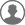注册

# 《原神》1.5原石快速获得攻略

• 编辑：小梅
2214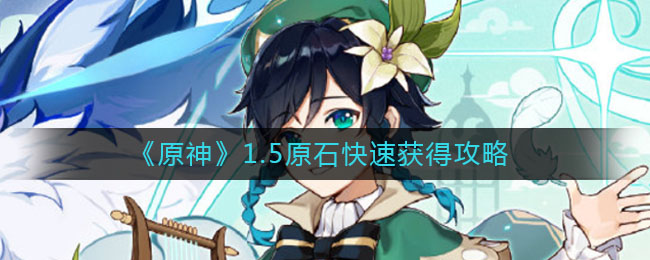### 《原神》1.5原石快速获得攻略

1.每天的委托任务 42*60= 2520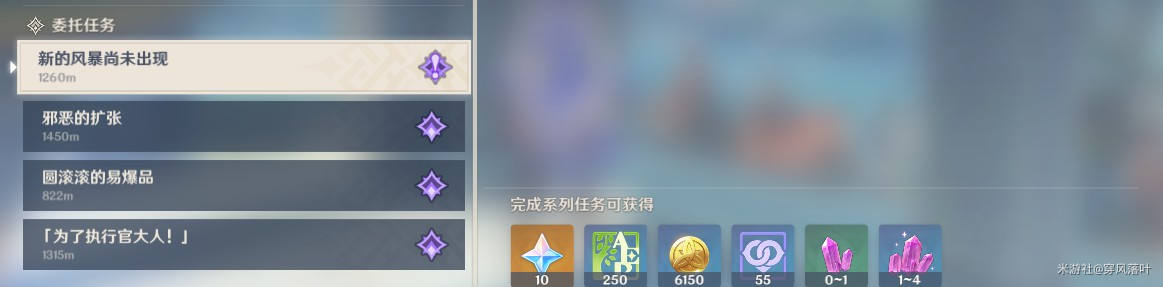2.两个角色钟离和优菈传说任务及卡池试用 60+60+20+20=1603.两个新邀约迪奥娜和诺艾尔 60+60=1204.家园体统 10级有60，如果每一级都有的话就是600，现在不算清楚，先10级估算一半奖励为300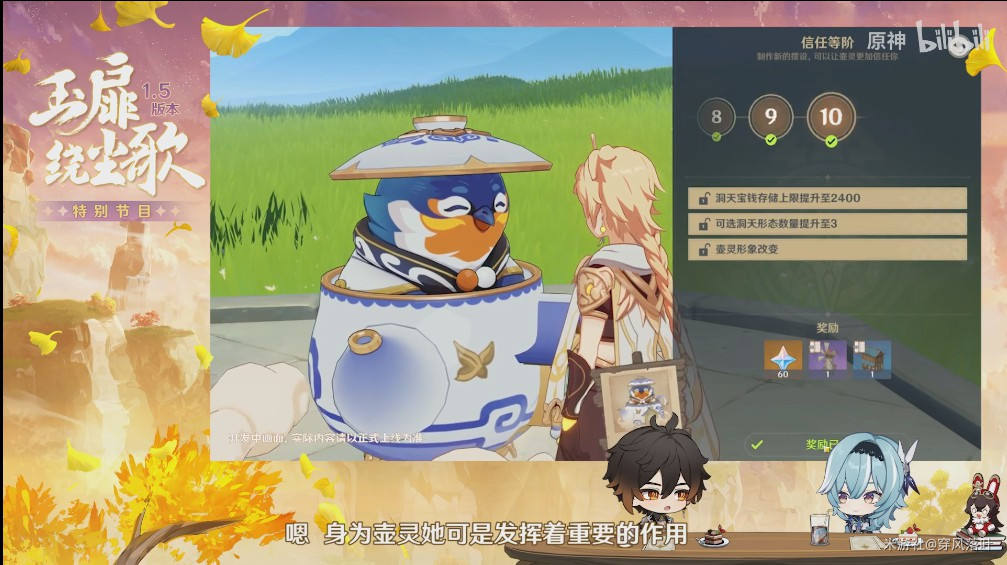5.成就具体更新未知数，但是多多少少的预计算个 100

6.导能圆盘·绪论(大世界收集，秘境挑战) 30一次，如果按照圆盘上的数目算的话，11个点就有有 330，现在也不算清楚， 也先估算6个点吧 30*6=180，然后4个秘境：4*60=240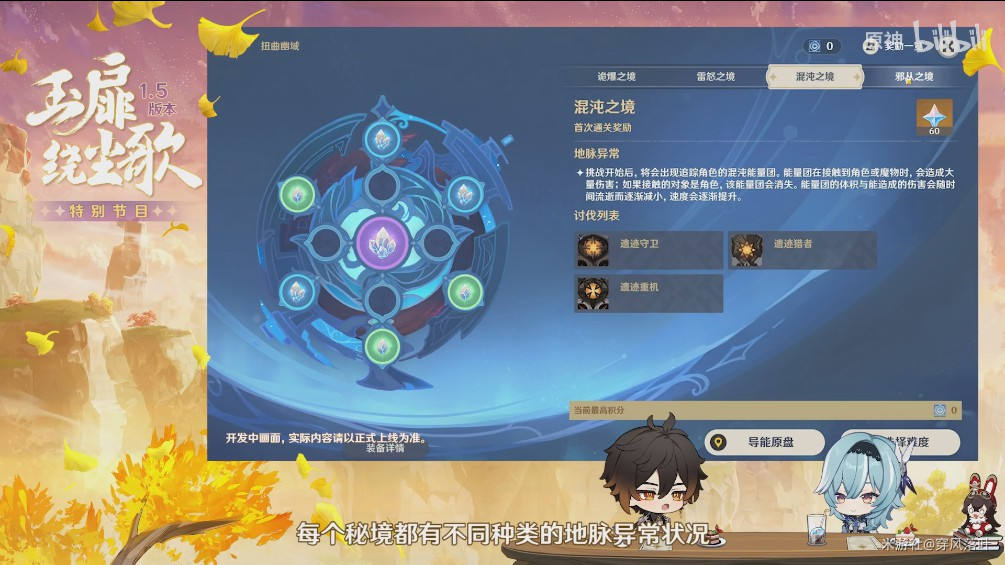7.迷城战线(限定角色通关秘境)有6关 6*70=420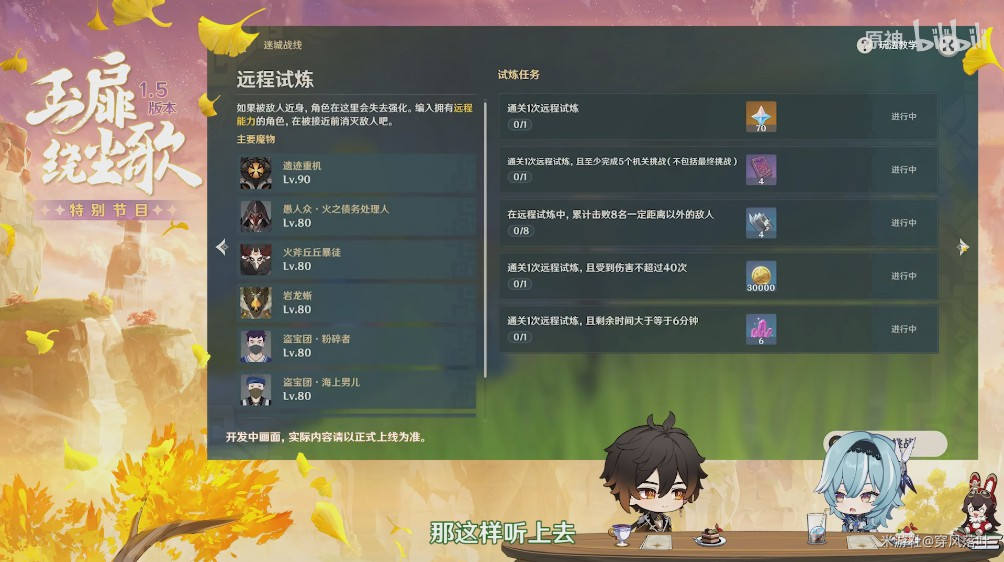8.折箭觅踪(追踪大伟丘)有7关根据以往相同任务的一关60的话 7*60=420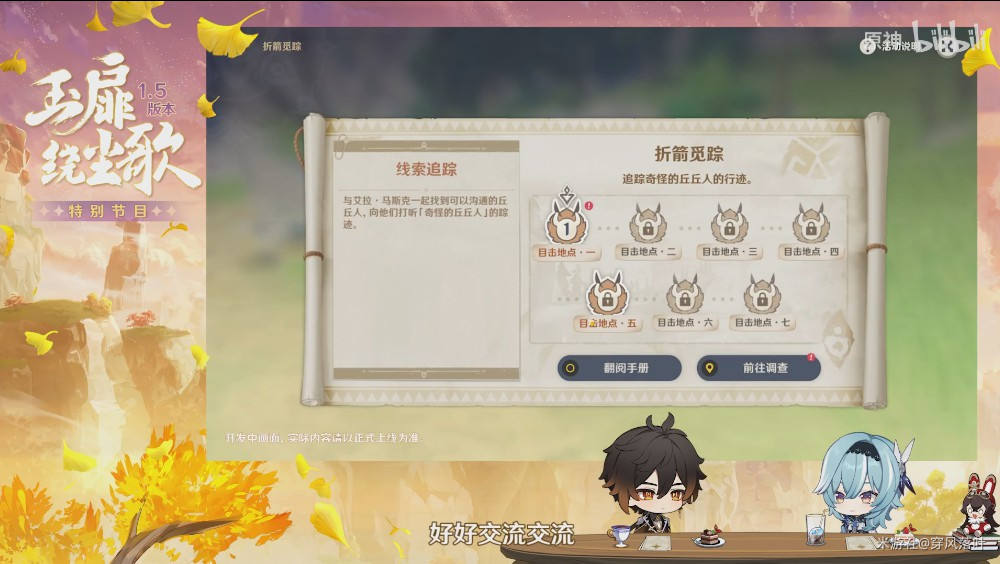9.风行迷踪 (躲猫猫)有5张地图 最终兑换预计 60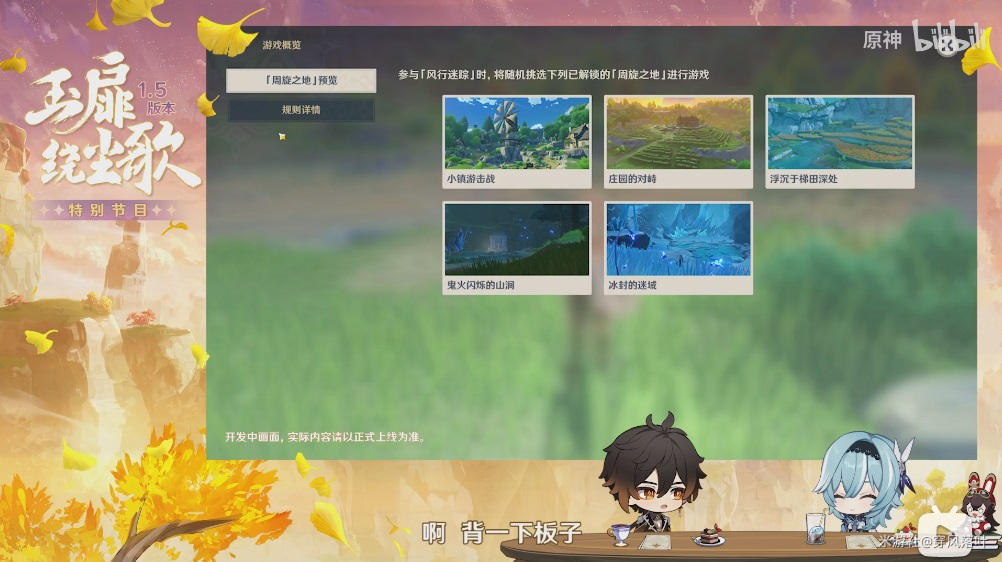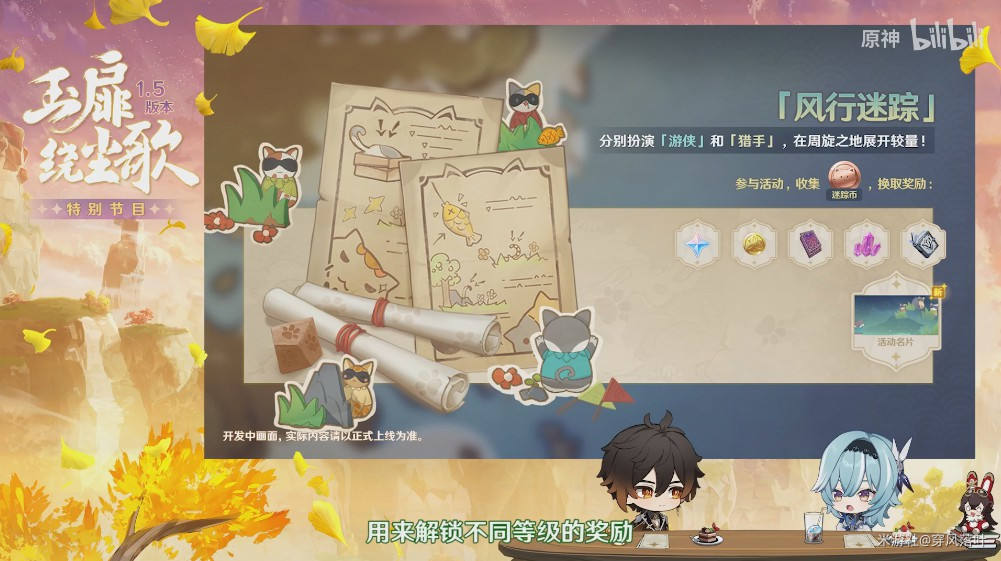10.维护更新 通常 300

11.更新后的7天签到活动 通常 300

12.深渊螺旋42天的话，三期 人均打满3*600=1800 保守点6星能过 1500

13.纪行满上50级免费相遇之缘 5=900

14.兑换纠缠之缘和相遇之缘42天在五月和六月 10+10=20=3200

15.米游社的签到 20+20+20=60

16.微博签到的 80

17.立本大爷 7*40=280+100(最终大奖)=380

18.月卡 90*42 =3780+300+300=4380

2520+160+120+300+100+420+420+420+60+300+300+1500+900+3200+60+80+380

=11240

11240+4380=15620食谱大全 up池更新顺序 隐藏宝箱位置 传说任务攻略汇总 稀有材料获取一览 游戏问题解决大全 公测角色一览 角色养成所需素材大全 世界任务攻略汇总 魔神任务攻略汇总 未归的熄星活动攻略汇总 书籍故事大全汇总 见闻奖励汇总一览 冒险家协会每日委托攻略汇总 全部商人NPC位置汇总 全成就攻略汇总主角 琴 安柏 丽莎 凯亚 芭芭拉 迪卢克 雷泽 温迪 可莉 班尼特 诺艾尔 菲谢尔 砂糖 莫娜 魈（未出） 北斗 凝光 香菱 行秋 重云 七七 刻晴 公子

6.3

3DM公众号

3DM自运营游戏推荐